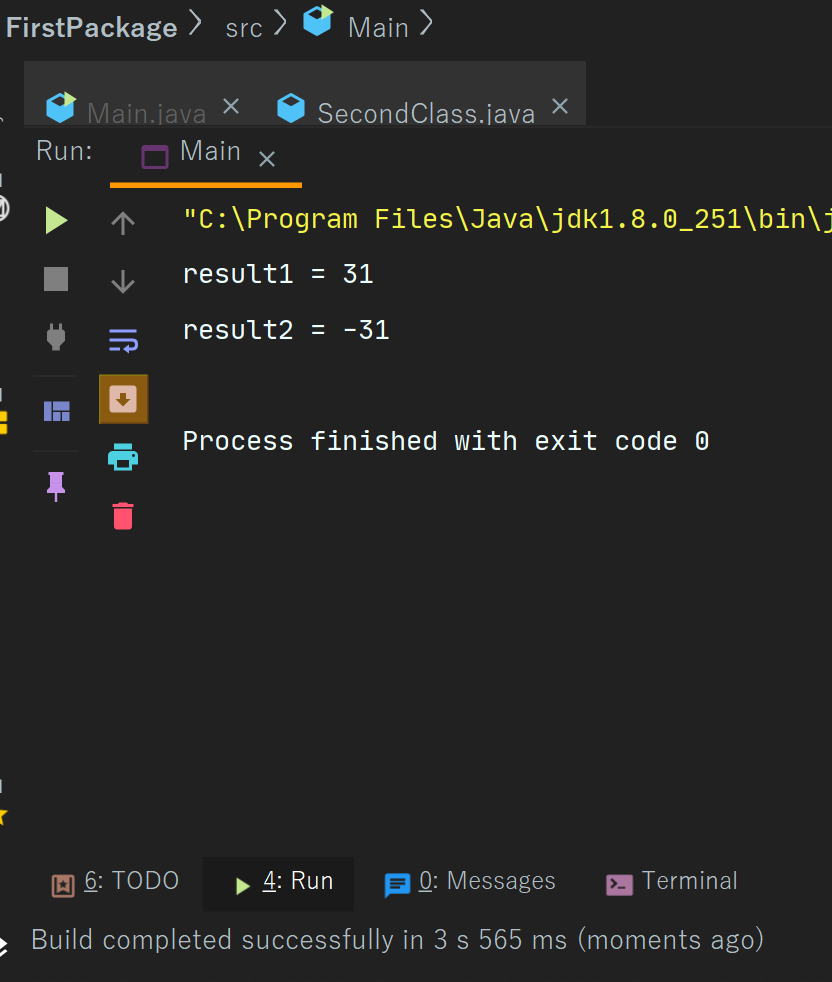Java Substraction Operators

# Java Substraction Operators

Subtraction Operator In Java
The subtraction operator in java is represented by minus sign which is indicated as  - “ - ”.
The subtraction operator is used for subtracting a value from another value.
It is also a part of the binary operator which means that this operator is used on two operators.
If we want to subtract any value by one then we can use the unary operator which is of two types we will see both of the types as follows  -
Decrement operator is of two types-
Post increment- In post decrement --  comes after the value like a--, or num- - .
In this operator, the value is firstly used and then decrement.
Pre decrement - In this operator -- comes before the value like  --a, --b, and the value is first decreased and then used.
Now we will see examples -
Example 1 -  here is a simple example of binary subtraction operator -
 import second.*; import java.util.*;   class Main{   public static void main(String[] args) {       int a = 54;       int b = 23;       int result1  = a-b;       int result2 = b-a;             System.out.println("result1 = "+result1);       System.out.println("result2 = "+result2);   }   }
Output -